﻿ Numerical Study of Density Functional Theory of Multi-electronic Atoms: Case of Carbon and HeliumPublications are Open
Access in this journal
Article Versions
Export Article
• Normal Style
• MLA Style
• APA Style
• Chicago Style
Original Article
Open Access Peer-reviewed

### Numerical Study of Density Functional Theory of Multi-electronic Atoms: Case of Carbon and Helium

Yande DIOUF , Kharouna TALLA, Saïdou DIALLO, Louis GOMIS
American Journal of Nanomaterials. 2021, 9(1), 12-22. DOI: 10.12691/ajn-9-1-2
Received February 21, 2021; Revised April 04, 2021; Accepted April 12, 2021

### Abstract

The ab-initio method based on density functional theory was used via local density approximation (LDA) to analyze the electronic structure of helium atoms and carbon. This involves calculating physical properties of these atoms such as effective potential, electron density and radial wave functions in both the ground state and the first three excited states. Thus, taking into account the structural complexity of the system, the calculations were carried out implicitly by the finite element method via the MATLAB software in deterministic mode. At the end of the study, the results obtained are consistent and revealing. They have shown that the effective potential of the helium atom decreases with position both in its ground state and in its first three excited states, so for the carbon atom, they have shown that these two states evolve in the same way. Regarding the electron density, the results revealed that at each radius of an atomic orbital, the electron density passes through a maximum for the helium atom while for carbon, the results show a succession of two and three peaks. Finally, with regard to radial wave functions, the results showed that the 1s orbital is closer to the nucleus than the 2s, 2p and 3s orbitals for the helium atom. For the carbon atom, the results confirm the information provided by the electron density. From the results it can be concluded that the effective potential of a multielectronic atom decreases with position and that the densest orbitals are those near the nucleus.

### 1. Introduction

During the last fifty years, solid-state physics has experienced an impressive boom both in the field of theory and in implementations of this theory 1, 2. It is about the application of quantum mechanics to molecular systems in order to extract the different physicochemical properties such as structural, elastic, thermal, magnetic, optical and electronic properties 3, 4. According to 5, 6, several formalisms or quantum approaches have been put in place to try to solve the problem. Mainly, we can distinguish three approaches: (i) the first purely empirical consisting in making experimental observations and carrying out measurements to obtain the values of the parameters which make it possible to describe the properties of the given system; (ii) the second semi-empirical consists in introducing a theoretical modeling but requires experimental studies to adjust the values of the modeling parameters and (iii) the third, the ab-initio approach, consists of starting from the fundamental laws of quantum physics and electromagnetism, and some basic characteristics defining the system to determine, explain or predict its structures and properties 7, 8. Among these approaches, the ab-initio approach has become a basic tool since the approach is to calculate the properties of complex systems from the fundamental laws governing the elementary constituents of these systems 9. This approach has now been able to replace experiments that are very expensive or even impractical in the laboratory or very dangerous 10. In ab-initio calculations, the approach consists in first determining the megatonic and ionic structures of the system and then deducing the values of the characteristic parameters 11, 12. This new method of calculation, which uses the computer tool, is based on density functional theory (DFT). It is a method which makes it possible to study the electronic structures of systems composed of several atoms and to deduce their physicochemical properties. Combined with numerical physics methods, DFT forms the basis of several of the most efficient and widely used ab-initio computational methods to determine the values of the parameters that define the properties of various materials and structures 13. DFT is derived from the work of 14, followed by that of 15. DFT makes it possible to tackle the problem of the electronic structure of ion-electron systems by using electron density as the main basic quantity 13, 14, 15, 16. The ab initio methods of numerical physics based on DFT have made it possible to obtain results that are interesting and difficult, if not impossible, to obtain with other approaches. The application of these methods and their extensions are particularly promising for nanosciences and nanotechnologies 17, 18. This is why interest in FTD and its applications is growing worldwide today 19, 20. It is in this same vein that the present study was initiated. This involves analyzing the electronic structure of multielectronic atoms such as Helium and Carbon through the ab-initio method which is based on the density functional theory using the local density approximation (LDA). This is a way of describing matter in its ground state no longer by going through the wave functions of the N electrons but rather by considering the electron density of these. This requires solving the Schrödinger equation, which is an N-body problem whose resolution is difficult, if not impossible, to obtain analytically 21. Thus, the putting into practice of ab-initio methods, such as those based on DFT, generally require numerical methods with adequate computer resources 1. These methods can therefore be considered to be within the framework of digital physics. The use of computer tools and methods, means that the modeling of real compounds (with possibly complex structures) becomes perfectly possible with means and within deadlines which remain "reasonable" and more precise, making it possible to obtain very quickly the results. properties of many solids 22, 23. It makes it possible to explain and predict the structures and properties of materials knowing a few parameters such as the atomic numbers of the constituent atoms 14, 15, 16, 17, 18, 19, 20, 21, 22, 23, 24. In practice, it allows the creation of a large number of materials currently used in industry and new technologies. In this study we used the MATLAB code in deterministic mode to do our calculations. The results obtained provided a better understanding of the organization of matter at the atomic scale in the case of helium and carbon. The choice of these atoms is no accident. It obeys well-defined rules: (i) they are atoms made up of several electrons; (ii) they have paramagnetic and ferromagnetism properties; (iii) their total energies calculated in their ground and excited states corroborate with those found by Slater's method and (v) several works in the literature testify to the targeting of these two atoms. Among these works, we can cite among others 25, 26, 27, 28 for the carbon atom and 29, 30, 31, 32, 33, 34 for the helium atom.

### 2. Materials and Methods

2.1. Problem Formulation

The density functional theory finds its roots in the Thomas-Fermi model which, from 1927, gives a very simple relation giving the total energy of an atomic or molecular system from its electron density alone (Parr and Yang, 1989). Then completed by Dirac who adds the exchange energy to it, this model suffers in the eyes of chemists from a congenital defect: the molecule is less stable than the atom. The first calculations attributable to the density functional theory date back to the Xα calculations introduced by Slater in 1951. However, the corresponding equations being obtained at the time by an approximation of the Hartree-Fock method, (also called ab initio by theoretical chemists), it did not seem conceivable that the Xα method could lead to better results than those obtained from the Hartree-Fock method 7. The density functional theory was in fact born in 1964 with the publication by Hohenberg and Kohn of their famous theorems: the first establishes, by reasoning by the absurd, that the ground state of an electronic system does not depend on than its sole density. It is only later that this theorem will be generalized to degenerate ground states, as well as to the excited states lowest in their class of symmetry. The second theorem is the analogue of the variational principle, and shows that the density is stationary for the ground state. But the existence of this stationary solution did not make it possible to make precise calculations, and it is the method proposed the following year by Kohn-Sham which will make possible calculations that are both less cumbersome than calculations by Hartree. -Fock, and with suitable precision: the originality of the Kohn-Sham method is to obtain the kinetic energy from orbitals representing a system of electrons without interactions having the same density, and which will obey to a Schrodinger-type equation 35. The energy of electronic interaction, which on the other hand is obtained by density alone, is divided into two contributions: classical Coulomb energy, and exchange-correlation energy. The latter in fact contains everything that is not contained in this kinetic energy KS and Coulomb energy, namely mainly the energy, which finds its origin in the quantum nature of electrons, the correlation energy, which translates the non-independence of electrons in their movements, as well as the difference between the kinetic energy calculated from orbitals representing a system without interaction and the real kinetic energy of the systems of interacting electrons 36.

2.2. DFT Formalism

The Theory of Functional Density has become, over the last decades, a theoretical tool that has taken a very important place among the methods used for the description and analysis of physical and chemical properties for complex systems, particularly for systems containing a large number of electrons 37. DFT is a reformulation of the N-body quantum problem and as the name suggests, it is a theory that only uses electron density as the fundamental function instead of the wave function as is the case in the method by Hartree and Hartree-Fock. The main goal of DFT is to replace the multielectronic wave function with electron density as the base quantity for calculations. While the multielectronic wave function depends on 3N variables (where N is the total number of particles in the system), the density is a function of three variables that it is an easier quantity to process as long as it is mathematically conceptually 4. The DFT allows us to solve the Schrödinger equation with N bodies by only involving the observable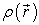defined in the physical space R3 which replaces a space of configurations with 3N variables in which is defined the wave function (Hartree-Fock) 38. The formalism of the DFT is based on the theorem of Hohenberg and Kohn. The most common version, the local density approximation (LDA), often gives results as good as those of Hartree-Fock-type methods, for less computation times. The Hamiltonian of a system of N electrons moving in a fixed external potential is given by equation (1) 39.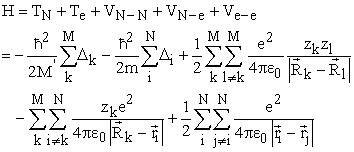(1)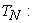kinetic energy of nuclei;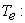kinetic energy of electrons;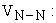potential nucleus-nucleus repulsion energy;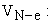potential electron-nucleus attraction energy;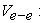electron-electron repulsion potential energy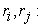electron positions 𝑖 𝑒𝑡 (𝑗) respectively.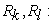positions of the nuclei (𝑘) 𝑒𝑡 (𝑙) respectively;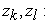atomic numbers of nuclei (𝑘) 𝑒𝑡 (𝑙) respectively;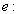electron charge; m: electron mass;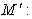core mass.

2.3. Basic Equations

Electrons are defined as indistinguishable and inseparable particles. In this reality, an electron cannot be located as much as an individual particle. Each particle has the probability of being present in a volume element. The electron densityis the probability of finding one of the N electrons in the element of volume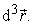Therefore, it is defined as the multiple integral on the space and spin coordinates of all electrons, represented by relation (2) 7.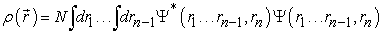(2)is a positive function depending only on the 3 coordinates (x, y, z) of space which tends to zero when r tends to infinity and whose integral over all space gives the number N of electrons.

2.3.1. Hohenberg and Kohn Equations

Once the electron density is defined, it is necessary to lay the foundations of the DFT, expressed for the first time by Hohenberg and Kohn in 1964 on which rests all the DFT which can be summed up in two theorems 14.

First theorem

The first theorem is used to establish a correspondence between the electron densityof a polyelectronic system in the ground state and the external potential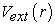of the nucleus so as to be able to describe the value of any observable as a unique functional of the exact electron density specific to this ground state. The consequence of this theorem is that the variation of the external potential then implies a variation of the electron density. It allows to define a universal functional of the electron density,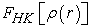called Hohenberg-Kohn functional, such that the energy of the atom is written according to equation (3) 7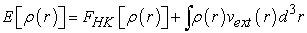(3)

whereis the unknown universal functional given by the relation (4)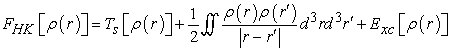(4)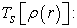Sum of the kinetic energies of the negatons;

The second term is the Coulomb interaction potential energy;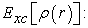exchange-correlation energy

Second theorem

The second theorem is a variational principle analogous to that proposed in the Hartree-Fock approach for a functional of the wave function, but applied this time to a functional of the electron density. This second theorem states that for any multi-electronic system with an electron number N and an external potential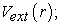the universal functional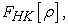expressing energy as a function of electron density, reaches its minimum value when the electron density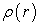corresponds to the exact density of the ground state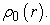Minimization of energy goes through the use of the Lagrange formalism, i.e., by establishing a Lagrangian given by equation (5) 40.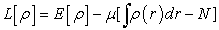(5)

where μ is the Lagrange multiplier; the minimization of the Lagrangian implies the relation (6):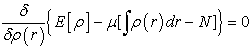(6)

As the differential of a functional is expressed by relation (7), we can thus rewrite equation (6) in the form of relation (8)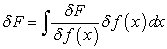(7)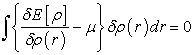(8)

This named relation, fundamental equation of the density functional theory, implies relations (9) and (10)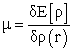(9)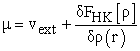(10)

2.3.2. Kohn-Sham Equations

Kohn and Sham assume that there is a fictitious system of N independent electrons with the same electron density as the real system of interacting electrons. The interest comes from the fact that the expressions of kinetic energy and potential energy for this system are known, the only indeterminate term is the exchange-correlation term. Then they proposed separations according to relation (11) 15.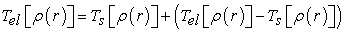(11)

where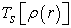is the kinetic energy of an electron gas without interaction which calculated by reintroducing an orbital description according to the relation (12)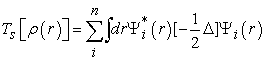(12)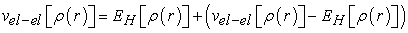(13)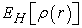is the Coulomb energy of electron-electron interaction, or the Hartree energy defined by equation (14):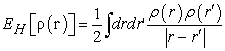(14)

The functional which is defined by relation (15) can also be written by relation (16)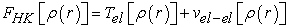(15)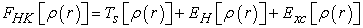(16)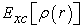is the correlation exchange term defined by relation (17)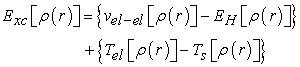(17)

This term includes the kinetic energy correlation for an interacting electron gas and the Hartree energy correction in the electron-electron interaction 26.

The density functional defined by equation (3) then relates to relation (18)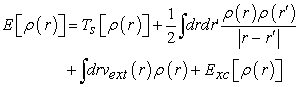(18)

Applying the variational principle to this functional leads to equation (19):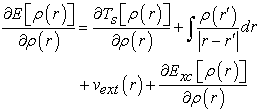(19)

Determining the ground state of the system then amounts to solving a set of equations called Kohn-Sham equations defined by relation (20) which can also be denied by equation (21) 41.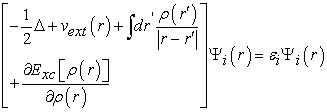(20)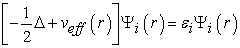(21)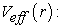effective potential is defined by relation (22)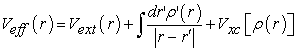(22)

The second term of this equality is the Hartree potential, which can be in the form of an average of an interaction potential given by equation (23):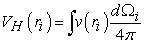(23)

Where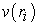is an interaction potential given by equation (24)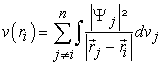(24)
2.4. Numerical Resolution

There are two ways of looking at an atomic system, either from its electron cloud via electron density, or through nuclei via external potential. Hohenberg and Khon have shown that there is a one-to-one correspondence between the external potential and the electron density, that is, one seems to be the image of the other. Thus, by knowing the electron density, the Hamiltonian operator can be determined and through the latter, the different properties of the molecule or material can be calculated 42. On the other hand, the fact that the total energy of a system in the ground state is a functional E [ρ (r)] of the electron density allowed Hohenberg and Khon to express this functional according to the expression (25) 7.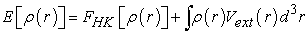(25)

Knowledge of the functionalmakes it possible to determine the total energy the charge density of the ground state for a given external potential, using the variational principle. Unfortunately, the theorem of Hohenberg and Khon does not give any indication of the form of and that this functionalremains unknown to this day. There is no exact formulation to express kinetic energy as an electron density functional. The equations of Khon and Sham present the only solution to this problem and which are established with the objective of providing the foundations necessary to effectively exploit the theorems of Hohenberg and Khon 43, 44. To do this, they introduced a further development which consists in replacing the real interactive system with a fictitious characterized in all respects by the same density as the system of interacting electrons. Schrödinger equation to be solved in the framework of the Kohn and Sham approach is of the following form (26) 45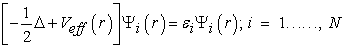(26)

Solving the Kohn and Sham equations requires the choice of a basis for the wave functions that can be taken as a linear combination of orbitals called Kohn-Sham orbitals (KS) written in the form defined by l'equation (27)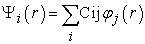(27)

Where the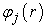are the basic functions and the Cij the expansion coefficients. Solving the Kohn and Sham equations comes down to determining the Cij coefficients for the occupied orbitals that minimize the total energy. This resolution is done numerically in an iterative manner following 6 steps 1:

i) We choose an initial density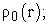ii) We determine the Kohn-Sham potential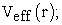iii) Solve the second Kohn-Sham equation giving the functions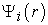and corresponding eigenvalues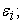iv) We calculate a new densityfrom the functions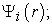v) We check the convergence criterion (by comparing the old and the new density). If the criterion is not yet satisfied, we start the cycle again. The most used convergence criteria are based on the difference between the energies or the densities corresponding to the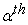et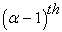iteration as shown in equation (28)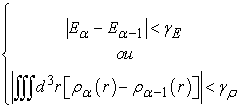(28)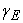and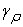being the values of the tolerances (precisions) that we choose from the start.

vi) Finally, the different physical quantities are calculated (Energy, forces, etc.); End of process.

All of these steps are shown schematically by the algorithm shown in Figure 1.

• Figure 1. Iterative process used in solving Kohn-Sham equations

Due to the structural complexity of the system, we have linearized the basic equations implicitly and solved numerically by the finite element method using Matlab software. MALAB (MATrix LABoratory) is programming software that makes it as easy as possible to transcribe a mathematical problem into computer language, using writing as close as possible to scientific natural language. A sequential resolution of the problem using this computer program, made it possible to quickly obtain interesting and difficult results, if not impossible, to obtain with other approaches such as the analytical one.

### 3. Results and Discussion

Below we present the graphical results relating to the effective potential, electron density and radial wave function for helium and carbon atoms.

• Figure 2. Variations depending on the position of the effective potential of the helium atom in its ground state (a) and its first three excited states (b, c, d)
• Figure 3. Variations depending on the position of the effective potential of the carbon atom in its ground state (a) and its first three excited states (b, c, d)
3.1. Effective Potential

In Figure 2 and Figure 3, we can see the variations in the effective potential as a function of the position for the helium and carbon atoms respectively in the ground state and in the first three excited states. Thus, the analysis in Figure 2 shows that there is a very rapid growth in the region of the heart represented here by the 1s orbital. In the outer region we noticed a slight increase in the effective potential. This allows us to conclude that the effective potential of the helium atom decreases with position. Reading Figure 3 shows that the effective potential of the carbon atom in its ground state as in excited states changes in the same way. It increases very rapidly in the area near the nucleus, but as we move away from the nucleus it begins to stabilize to tend towards a limit value for all four states studied.

• Figure 4. Variations depending on the position of the electron density of the helium atom in its ground state (a) and its first three excited states (b, c, d)
• Figure 5. Variations depending on the position of the electron density of the carbon atom in its ground state (a) and its first three excited states (b, c, d)
3.2. Electron Density

Figure 4 and Figure 5 illustrate the evolution of electron density following the position of the helium and carbon atoms in the ground state and in the first three excited states, respectively. The analysis of Figure 4 shows that at each radius of an atomic orbital, the electron density passes through a maximum. In the ground state all the electrons are in the 1s orbital, this gives a very dense orbital characterized by a maximum of the electron density higher than all the maxima found for this orbital in the excited states. At the excited states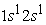and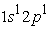we have two electron density maxima; the electrons have reached the radii of the 1s and 2s orbitals. In its states the 1s orbital is denser than the 2s and 2p orbitals and has a maximum electron density much greater than the maximum in the 2s or 2p orbital. In the excited state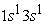the 3s orbital is small compared to the 1s orbital which is larger and closer to the nucleus. The electron density in this state goes through a maximum at the radius of the 1s orbital, at the radius of the 3s orbital we also have a maximum of the very small electron density before the maximum found at the radius of the 1s orbital. The analysis of Figure 5 in the four states studied shows a succession of two and three peaks. In the ground state and in the excited state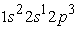we have two electron density maxima, the electrons have reached the radii of the 1s, 2s and 2p orbitals. The maximum electron density at the smallest position is greater than the other maxima. The 1s orbital is denser than the 2s and 2p orbitals. In the excited states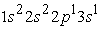and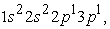we have three maxima. The third maximum appears at the radius of the 3s orbital.

In Figure 6 and Figure 7, we have a presentiment of the variations of the radial wave functions as a function of the position for the helium and carbon atoms respectively in the ground state and in the first three excited states. This is to describe the regions of space where the electrons of helium and carbon atoms are likely to be found. Analysis of Figure 6 shows that the 1s orbital is closer to the nucleus than the 2s, 2p and 3s orbitals. The 2s and 2p orbitals have their electrons farther from the nucleus than those of the 1s orbital but closer than the electron in the 3s orbital. The analysis of Figure 7 confirms information provided by the electron density. It also shows the negative part of the radial wave function.

• Figure 6. Variations depending on the position of the radial wave functions of the helium atom in its ground state (a) and its first three excited states (b, c, d)
• Figure 7. Variations depending on the position of the radial wave functions of the carbon atom in its ground state (a) and its first three excited states (b, c, d)

### 4. Conclusion

Nowadays, the density functional theory has established itself as a relatively fast and reliable way to simulate the electronic and structural properties for all the elements of the periodic table. In this work, we are interested in the study of the electronic structure of helium and carbon atoms in the approximation of the density functional theory. To this end, we have developed a MATLAB code based on this same theory to determine physical quantities such as the effective potential, the electron density and the radial wave functions of these atoms in their ground state and certain excited states. At the end of the study, the results obtained are consistent and revealing. For the helium atom, there is a very rapid growth of the effective potential in the region of the heart represented here by the 1s orbital. In the outer region we noticed a slight increase in the effective potential. This allows us to conclude that the effective potential of the helium atom decreases with position. For the carbon atom, the effective potential in its ground state as in excited states, evolves in the same way. There is a very rapid increase in potential in the area near the nucleus, but as we move away from the nucleus, it begins to stabilize to tend towards a limit value for all four states studied. Regarding the electron density, we note that in the ground state all the electrons are in the 1s orbital, this gives a very dense orbital characterized by a maximum greater than all the maxima found for this orbital in the excited states. At the excited statesand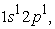we have two maxima, the electrons have reached the radii of the 1s and 2s orbitals. In these states the 1s orbital is denser than the 2s and 2p orbitals and has a much higher maximum than that obtained in the 2s or 2p orbital. In the excited statethe 3s orbital is small compared to the 1s orbital which is larger and closer to the nucleus. The electron density in this state passes through a maximum at the radius of the 1s orbital; at the radius of the 3s orbital we also have a very small maximum compared to that found at the radius of the 1s orbital. Regarding the carbon atom, we note that in the ground state and in the excited state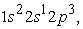we have two maxima of the electron density, the electrons reached the radii of the 1s, 2s and 2p orbitals. The maximum electron density at the smallest position is greater than the other maxima. The 1s orbital is denser than the 2s and 2p orbitals. In the excited statesandwe have three maxima. The third maximum appears at the radius of the 3s orbital. Finally, with respect to radial wave functions, it is a question of describing the regions of space where the electrons of helium and carbon atoms are likely to be found. Thus, we note that for the helium atom, the 1s orbital is closer to the nucleus than the 2s, 2p and 3s orbitals. The 2s and 2p orbitals have their electrons farther from the nucleus than those of the 1s orbital but closer than the electron in the 3s orbital. For the carbon atom, the results confirm the information provided by its electron density. They also show the negative part of the radial wave function. Ultimately, we conclude that the effective potential of a multielectronic atom decreases with position and that the densest orbitals are those near the nucleus. The density functional theory is therefore a good approximation for the treatment of the electronic structure of multielectronic atoms. However, to give this study all its quintessence, it is imperative to validate these results by exploiting other ab-initio methods such as the linearized augmented plane wave method (FP-LAPW) and the linearized muffin-tin orbitals method (FP-LMTO).

### Acknowledgements

This study is part of our research work on a unique doctoral thesis from Cheikh Anta Diop University (Senegal). It received no financial support except for our meager means. However, on a scientific level, we have benefited from the expertise of Dr V.B. TRAORE. To this end, we thank him for his spirit of sharing.

### Competing Interests

The authors declare no competing interests.

### References

  Bahnes, A., Vos, W., L., Kuijper, A., Barrat, J., L., and chouten, J., A., “High pressure phase diagram of helium hydrogen calculated through fluid integral equations and density functional theory of freezing’’, J. Phys. Cond. Matt., 3.11.1613.1991 “Study of two first-principle methods applied to Heuslers”, Master's Thesis, USTO-MB, Algerie .128p, 2014. In article View Article  Zuehlsdorff, T., J., and al., “Linear-scaling time-dependent density-functional theory in the linear response formalism” J. Chem. Phys. 139. 064104. 2013. In article View Article  PubMed  Holzapfel, W., B, “Physics of solids under strong compression”, Reports on Progress in Physics, 59. 29-9. 1996. In article View Article  Kohn, W., “Nobel Lecture: Electronic structure of matter - wave functions and density functionals”, Rev. Mod. Phys 71.1253. 1999. In article View Article  Diola, B., “Understanding density functional theory (DFT) and completing it in practice”, AIP Advances 4, 127104. 2014. In article View Article  Ravo, T., R., Raoelina, A., Hery, and A., Rakotoson, H., “The Functional Theory of Density and its applications in Nanotechnology” communication made during the first international conference on Nanotechnology in Madagascar, April 11-12, 2018. In article  Kohn, W., Becke, A., D., and Parr, R., G., “Density Functional Theory of Electronic Structure”, J. Phys. Chem. 100. 31. 12974-1298. 1996. In article View Article  Zhibing, Li. “Density functional theory for field emission from carbon nano-structures”, 159, Part 2, 162-172. 2015. In article View Article  PubMed  Gonze, X., Rignanese, G., M., Verstraete, M., Beuken, J., M., Pouillon, Y., Caracas, R., Jollet, F., Torrent, M., Zerah, G., Mikami, M., Ghosez, Ph., Veithen, M., Raty, J., Y., Olevano, V., Bruneval, F., Reining, L., Godby, R., Onida, G., Hamann, D., R. and Allan., D., C., “A brief introduction to the ABINIT software package”, Z. Kristallogr, 220. 558-562. 2005. In article  Hafner, J., Wolverton, C., Ceder, G., “Toward computational material design: The impact of Density Functional Theory on material research”, MRS Bull, 31. 681. 2006. In article View Article  Ziegler, T., “Approximate density functional theory as a practical tool in molecular energetics and dynamics”, Chem. Rev. 91. 651-667. 1991. In article View Article  Yang, Z., H., Pribram, J., A., Burke, K., and Ullrich, C., A., “Direct extraction of excitation energies from ensemble density-functional theory”, Phys. Rev. Lett. 119. 033003. 2017. In article View Article  PubMed  Gonze, X., Amadon, B., Anglade, P., M., Beuken, J., M., Bottin, F., Boulanger, P. , Bruneval, F., Caliste, D., Caracas, R., Cote, M., Deutsch, T., Genovese, L., Ghosez, Ph., Giantomassi, M., Goedecker, S., Hamann, D., R., Hermet, P., Jollet, F., Jomard, G., Leroux, S., Mancini, M., Mazevet, S., Oliveira, M., J., T., Onida, G., Pouillon, Y., Rangel, T., Rignanese, G., M., Sangalli, D., Shaltaf, R., Torrent, M., Verstraete, M., J., Zerah, G., and Zwanziger, J., W., “ABINIT: First-principles approach of materials and nanosystem properties”, Computer Phys. Comm, 180. 2582-2615. 2009. In article View Article  Hohenberg, P., Kohn, W., “Inhomogeneous Electron Gas”, Physical Review, 136. 3B. B864-B871. 1964. In article View Article  Kohn, W., Sham, L., J., “Self-Consistent Equations Including Exchange and Correlation Effects”, Physical Review, 140. 4A. A1133-A1138. 1965. In article View Article  Blackburn, S., “Analysis of the electronic properties of superconductors using the density functional theory”, Thesis, University of Montreal, 2013. In article  Serdar, Ö., “First principles Modeling of Nanostructures”, Turk J Phys. 27. 2003. In article  Tang, Q., Zhen, Z., Zhongfang, C., “Innovation and discovery of graphene-like materials via density-functional theory computations”, WIREs Comput Mol Sci, 5. 360-379. 2015. In article View Article  Shamim, M., D., and Harbola, M., K., “Application of an excited state lda exchange energy functional for the calculation of transition energy of atoms within time independent density functional theory”, Journal of Physics B: Atomic, Molecular and Optical Physics. 43. 215002. 2010. In article View Article  Jones, R., O., “Density functional theory: Its origins, rise to prominence, and future”, Reviews of Modern Physics, 87. 2015. In article View Article  Yousef, S., James, R., C., Suzanne, M., S., “Numerical methods for electronic structure calculations of materials”, SIAM Review, 52.1. 3-54. 2010. In article View Article  Evangelista, F., A., Shushkov, P., and Tully, J., C. “Orthogonality constrained density functional theory for electronic excited states”, The Journal of Physical Chemistry A 117. 7378. 2013. In article View Article  PubMed  Xiao, D., W., Richard, L., M., Thomas, M., H., and Gustavo, E., S., “Density Functional Theory Studies of the Electronic Structure of Solid State Actinide Oxides”. Chem. Rev, 113. 2. 1063-1096. 2013. In article View Article  PubMed  Gonze, X., Beuken, J., M., Caracas, R., Detraux, F., Fuchs, M., Rignanese, G., M., Sindic, L., Verstraete, M., Zerah, G., Jollet, F., Torrent, M., Roy, A., Mikami, M., Ghosez, Ph., Raty, J., Y., and Allan., D., C., “First-principles computation of material properties: the ABINIT software project”, Computational Materials Science. 25. 478-492. 2002. In article View Article  Novoselov K., S., “Electric Field Effect in Atomically Thin Carbon Films”, Science.306.5696. 666-669. 2004. In article View Article  PubMed  Hughes, G., Thomas, J., Marsh, H., and Reed, R., “Origin of etch pits on graphite surfaces”, Carbon 1. 339-343.1964. In article View Article  McKee, D., W., “Metal oxides as catalysts for the oxidation of graphite”. Carbon 8, 131. 1970. In article View Article  Harris, P., “Controlled atmosphere electron microscopy studies of graphite gasification-4. Catalysis of the graphite-O2 reaction by silver”. Carbon 12, 189-197. 1974. In article View Article  Laulan, M., “Doctoral thesis in Nuclei, Atoms, Aggregates, Plasma,” Study of helium and beryllium atoms in an intense and short laser field”, University of Bordeaux I, 2004. In article  Militzer, B., “Correlations in hot dense helium”, J. Phys. A.Math. Theor., 42.21. 214001. 2009. In article View Article  Nettelmann, N., Holst, B., Kietzmann, A., French, M., Redmer, R., and Blaschke, D, “Ab Initio Equation of State Data for Hydrogen, Helium, and Water and the Internal Structure of Jupiter”, Astrophys. J., 683. 1217, August. 2008. In article View Article  Kowalski, P., M., Mazevet, S., Saumon, D., and Challacombe, M, “Equation of state and optical properties of warm dense helium”, Phys. Rev. B.76.075112.Aug. 2007. In article View Article  Lorenzen, W., Holst, B., and Redmer, R., “Demixing of hydrogen and helium at megabar pressures”, Phys. Rev. Lett., 102: 115701, Mar. 2009. In article View Article  PubMed  Vos, W., L., Kuijper, A., Barrat, J., L., and chouten, J., A., “High pressure phase diagram of helium hydrogen calculated through fluid integral equations and density functional theory of freezing”, J. Phys. Cond. Matt., 3.11.1613. 1991. In article View Article  Ayers, P., W., Levy, M., and Nagy, “Communication: Kohn-Sham theory for excited states of coulomb systems”, The. Journal of Chemical Physics 143. 191101. 2015. In article View Article  PubMed  Ayers, P., W., Levy, M., and Nagy, A., “Time-independent density-functional theory for excited states of Coulomb systems”, Phys. Rev. A 85. 042518. 2012. In article View Article  Subhayan, R., Stefano, S., and David D., O., “Neutral excitation densityfunctional theory: an efficient and variational first-principles method for simulating neutral excitations in molecules”, Scientific Reports, 10.8947. 2020. In article View Article  PubMed  Zexing, Q., Yingjin, M., “Variational Multistate Density Functional Theory for a Balanced Treatment of Static and Dynamic Correlation”. Journal of Chemical Theory and Computation, 16. 8. 4912-4922. 2020. In article View Article  PubMed  Krykunov, M., Grimme, S., and Ziegler, T., “Accurate theoretical description of the 1la and 1lb excited states in acenes using the all order constricted variational density functional theory method and the local density approximation”, J. Chem. Theory Comput. 8. 4434-4440. 2012. In article View Article  PubMed  Kohn, W., Becke, A., D., and Parr, R., G., “Density Functional Theory of Electronic Structure”, J. Phys. Chem. 100.31. 12974-1298. 1996. In article View Article  Kowalczyk, T., Tsuchimochi, T., Chen, P., T., Top, L., and Voorhis, T., V., “Excitation energies and Stokes shifts from a restricted open-shell Kohn-Sham approach”, J. Chem. Phys, 138. 164101. 2013. In article View Article  PubMed  Ruiz, S., M., Skylaris, C., K., A., “variational method for density functional theory calculations on metallic systems with thousands of atoms”, J. Chem. Phys. 139.054107. 2013. In article View Article  PubMed  Hanson-Heine, M., W., D., George, M., W., and Besley, N., A., ”Calculating excited state properties using Kohn-Sham density functional theory”, J. Chem. Phys. 138.064101. 2013. In article View Article  PubMed  Seidl, A., Görling, A., Vogl, P., Majewski, J., A., Levy, M., “Generalized Kohn-Sham schemes and the band-gap problem”, Phys. Rev. B. 53.3764-377. 1996. In article View Article  PubMed  Parr, R., G., Yang, W., ”Density-Functional Theory of Atoms and Molecules”, Oxford Uni-versity Press, Oxford, 1989. In article

Published with license by Science and Education Publishing, Copyright © 2021 Yande DIOUF, Kharouna TALLA, Saïdou DIALLO and Louis GOMISThis work is licensed under a Creative Commons Attribution 4.0 International License. To view a copy of this license, visit http://creativecommons.org/licenses/by/4.0/